# Gas Temperature Volume and PressurePage 1

#### WATCH ALL SLIDES

Slide 1Gas Temperature, Volume, and Pressure

This tool can measure the temperature, volume, and pressure of a gas

Slide 2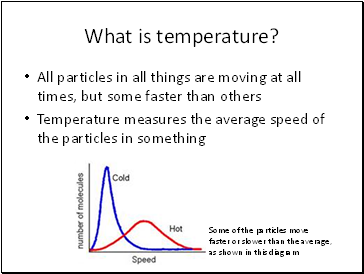## What is temperature?

All particles in all things are moving at all times, but some faster than others

Temperature measures the average speed of the particles in something

Some of the particles move faster or slower than the average, as shown in this diagram

Slide 3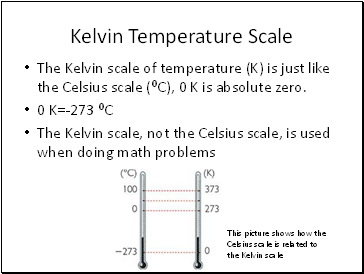## Kelvin Temperature Scale

The Kelvin scale of temperature (K) is just like the Celsius scale (⁰C), 0 K is absolute zero.

0 K=-273 ⁰C

The Kelvin scale, not the Celsius scale, is used when doing math problems

This picture shows how the Celsius scale is related to the Kelvin scale

Slide 4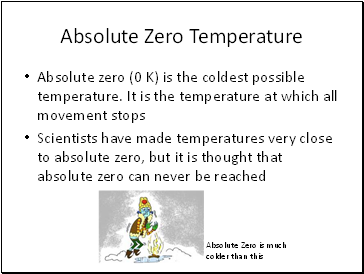## Absolute Zero Temperature

Absolute zero (0 K) is the coldest possible temperature. It is the temperature at which all movement stops

Scientists have made temperatures very close to absolute zero, but it is thought that absolute zero can never be reached

Absolute Zero is much colder than this

Slide 5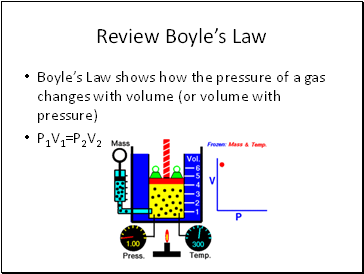## Review Boyle’s Law

Boyle’s Law shows how the pressure of a gas changes with volume (or volume with pressure)

P1V1=P2V2

Slide 6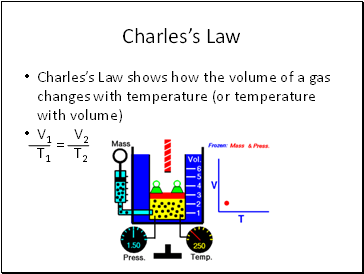## Charles’s Law

Charles’s Law shows how the volume of a gas changes with temperature (or temperature with volume)

V1 V2

T1 T2

Slide 7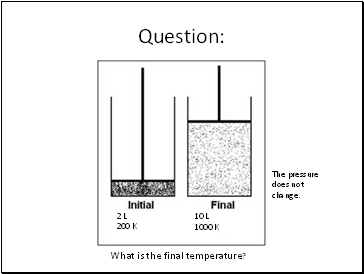Question:

2 L

200 K

10 L

What is the final temperature?

1000 K

The pressure does not change.

Slide 8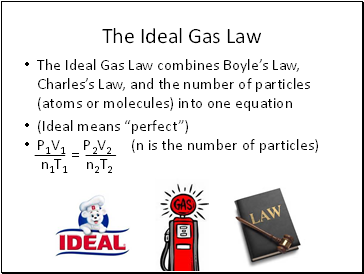## The Ideal Gas Law

The Ideal Gas Law combines Boyle’s Law, Charles’s Law, and the number of particles (atoms or molecules) into one equation

(Ideal means “perfect”)

P1V1 P2V2 (n is the number of particles)

n1T1 n2T2

Slide 9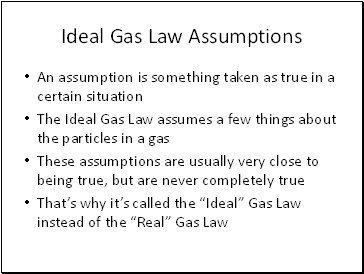## Ideal Gas Law Assumptions

An assumption is something taken as true in a certain situation

The Ideal Gas Law assumes a few things about the particles in a gas

These assumptions are usually very close to being true, but are never completely true

That’s why it’s called the “Ideal” Gas Law instead of the “Real” Gas Law

Slide 10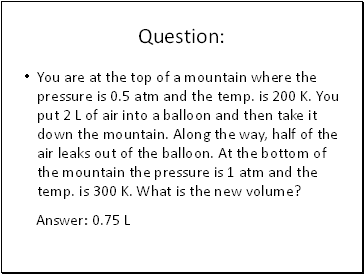Go to page:
1  2